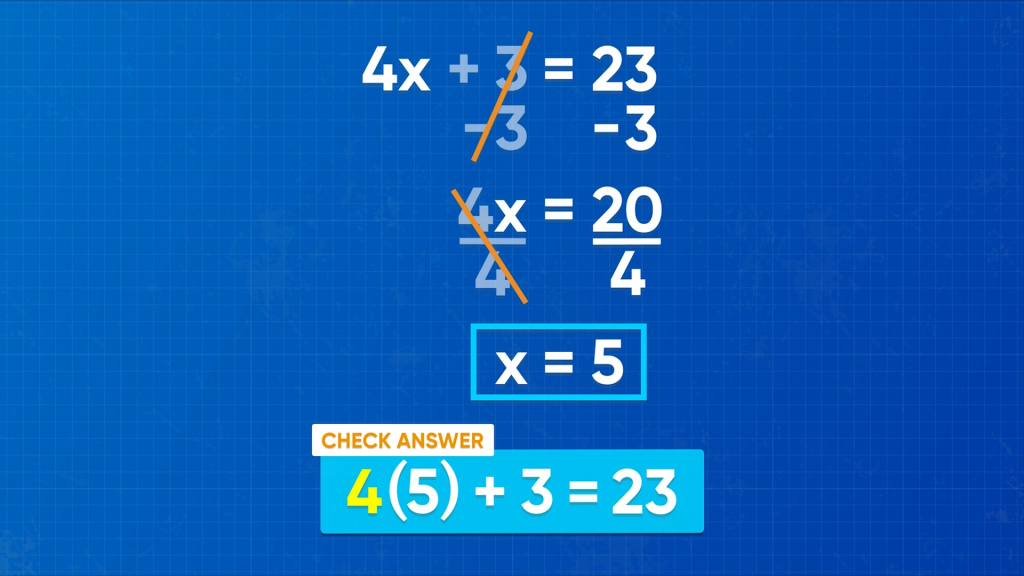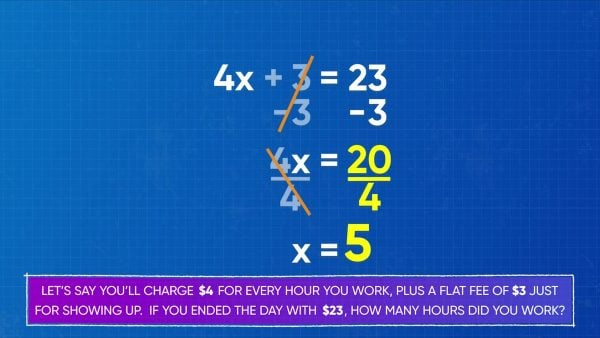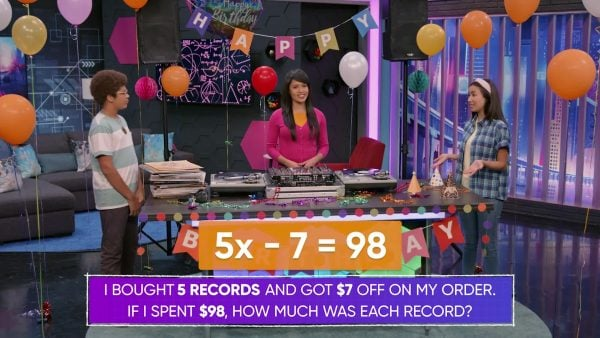Read about Solving Algebraic Equations (2-Step) | for kids Grade 6, 7, & 8
1%
It was processed successfully!WHAT ARE 2-STEP ALGEBRAIC EQUATIONS?

Students learn to find all factor pairs for a whole number in the range 1-100. Students will also recognize that a whole number is a multiple of each of its factors. They will determine whether a given whole number in the range 1-100 is a multiple of a given one-digit number and whether a given whole number in the range 1-100 is prime or composite.

To better understand 2-step algebraic equations…

WHAT ARE 2-STEP ALGEBRAIC EQUATIONS?. Students learn to find all factor pairs for a whole number in the range 1-100. Students will also recognize that a whole number is a multiple of each of its factors. They will determine whether a given whole number in the range 1-100 is a multiple of a given one-digit number and whether a given whole number in the range 1-100 is prime or composite. To better understand 2-step algebraic equations…

## LET’S BREAK IT DOWN!

### Solve equations with addition and multiplication.To rake leaves for your neighbors, you charge \$4 for every hour you work, plus a flat fee of \$3 for showing up. If you raked one lawn for \$23, how many hours did you work? We can represent this using an equation, if we let x be the number of hours worked. We write 4x + 3 = 23. To get x on its own, we need to get rid of everything else on the left side. We can do that by using inverse operations. The inverse of + 3 is – 3, so we subtract 3 on both sides. Then we have 4x = 20. Next, 4x means 4 times x, and the inverse is to divide by 4. So we divide both sides by 4, and then we have x = 5. You worked for 5 hours! Now you try: Find x if 3x + 5 = 17.

Solve equations with addition and multiplication. To rake leaves for your neighbors, you charge \$4 for every hour you work, plus a flat fee of \$3 for showing up. If you raked one lawn for \$23, how many hours did you work? We can represent this using an equation, if we let x be the number of hours worked. We write 4x + 3 = 23. To get x on its own, we need to get rid of everything else on the left side. We can do that by using inverse operations. The inverse of + 3 is – 3, so we subtract 3 on both sides. Then we have 4x = 20. Next, 4x means 4 times x, and the inverse is to divide by 4. So we divide both sides by 4, and then we have x = 5. You worked for 5 hours! Now you try: Find x if 3x + 5 = 17.

### How can we check our work?We solved 4x + 3 = 23, and found that x = 5. If we want to check our answer, we can do that by substituting the answer into the original equation. Now that we know that x = 5, we can substitute 5 for x in the equation, so that we get 4(5) + 3 = 23. 4 times 5 is 20, and adding 3 to that is 23. Since both sides of the equal sign have the same value, we know that our work checks out! Now you try: Check if x = 2 is true for 6x + 4 = 16.

How can we check our work? We solved 4x + 3 = 23, and found that x = 5. If we want to check our answer, we can do that by substituting the answer into the original equation. Now that we know that x = 5, we can substitute 5 for x in the equation, so that we get 4(5) + 3 = 23. 4 times 5 is 20, and adding 3 to that is 23. Since both sides of the equal sign have the same value, we know that our work checks out! Now you try: Check if x = 2 is true for 6x + 4 = 16.

### Solve equations with multiplication and subtraction.You got a deal at the local record store. You bought 5 records and got \$7 off. If your total came to \$98, how much was each record, assuming they all cost the same amount? If we let x be the price of a record, our equation becomes 5x – 7 = 98. First we want to get rid of – 7, and the inverse operation to subtraction is addition. So, we add 7 on both sides. Now we have 5x = 105. If we want to have only one x on the left side, we can divide both sides by 5. We get x = 21. So, each record cost \$21! Now you try. Find x if 3x – 7 = 29.

Solve equations with multiplication and subtraction. You got a deal at the local record store. You bought 5 records and got \$7 off. If your total came to \$98, how much was each record, assuming they all cost the same amount? If we let x be the price of a record, our equation becomes 5x – 7 = 98. First we want to get rid of – 7, and the inverse operation to subtraction is addition. So, we add 7 on both sides. Now we have 5x = 105. If we want to have only one x on the left side, we can divide both sides by 5. We get x = 21. So, each record cost \$21! Now you try. Find x if 3x – 7 = 29.

### Solve two-step equations with fractions.You took out one third of the money in your piggy bank, and you already have \$8 in your pocket. If the total amount of money you have now is \$16, how much was in the piggy bank? If we let x be the amount of money in the piggy bank, then our equation is [ggfrac]x/3[/ggfrac]+8=16. Remember that the line between x and 3 means division. To solve for x, first we subtract 8 from both sides, and now we have [ggfrac]x/3[/ggfrac]=8. Since x is divided by 3, the inverse operation is multiplication, so we multiply both sides by 3 to get x alone. Now we have x=24. That means that originally you had \$24 in the piggy bank! Now you try: Find x if [ggfrac]x/5[/ggfrac]+2=22.

Solve two-step equations with fractions. You took out one third of the money in your piggy bank, and you already have \$8 in your pocket. If the total amount of money you have now is \$16, how much was in the piggy bank? If we let x be the amount of money in the piggy bank, then our equation is [ggfrac]x/3[/ggfrac]+8=16. Remember that the line between x and 3 means division. To solve for x, first we subtract 8 from both sides, and now we have [ggfrac]x/3[/ggfrac]=8. Since x is divided by 3, the inverse operation is multiplication, so we multiply both sides by 3 to get x alone. Now we have x=24. That means that originally you had \$24 in the piggy bank! Now you try: Find x if [ggfrac]x/5[/ggfrac]+2=22.

## WHAT ARE 2-STEP ALGEBRAIC EQUATIONS VOCABULARY

Variable
A symbol, like x, that represents an unknown quantity.
Equals Sign
Tells us that the expressions on either side have the same value.
Equation
Two expressions that are equal to each other separated by an equal sign.
Algebraic Expression
An equation that uses one or more variables.
One-step equation
An equation that requires only one operation to isolate the variable.
Two-step equation
An equation that requires two operations to isolate the variable.
Operations that do the opposite of each other. Addition and subtraction are inverse operations, and multiplication and division are inverse operations.
To multiply the number in front of an expression in parentheses by everything inside the parentheses.

## WHAT ARE 2-STEP ALGEBRAIC EQUATIONS DISCUSSION QUESTIONS

### What are the operations in the equation 4x – 5 = 7? What operations do you need to use to solve for x?

The operations are multiplication and subtraction. So to solve for x, I need to use the inverse operations: division and addition.

### To solve 4x – 5 = 7, should you divide first or add first?

I could do either first, but if I divide first, I have to divide 5 by 4 and 7 by 4, which means that I have to work with fractions. That would take longer but still get the right answer. However, if I add 5 to both sides first, then the rest of the solution is much easier.

### How can you solve x3-2=2?

[ggfrac]x/3[/ggfrac] means x divided by 3, so the inverse operation is multiplication. I also need to add to isolate the variable. It is simpler to first add 2 on both sides, and then to multiply both sides by 3, but working in the opposite order would also result in the same answer. Then x= 12.

### What are two different ways you can solve 2(x – 3) = 8?

Method 1: Distribute 2 across the expression in the parentheses, so that I have 2x – 6 = 8, and then solve by adding 6 to both sides, and then dividing both sides by 2. Method 2: First divide both sides by the factor 2, so that I have x – 3 = 4. Then solve by adding 3 to both sides. Method 2 is more efficient.

### To solve 2x + 3 = 5, Sylvia first subtracted 5 from both sides. What did she do wrong?

Because Sylvia did the same operation to both sides, the equation is still correct: the two sides still equal. However, she is no closer to finding out the value of x. She should instead subtract 3 on both sides, which gets her closer to having x alone on the left side.
X

## Success

We’ve sent you an email with instructions how to reset your password.
Ok